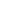# Use the associative property to subtract from three-digit numbers and verify solutions with addition

EngageNY 60 min(s)
In this lesson, students use the compensation strategy--an application of the associative property--to subtract from three-digit numbers. They learn to add or subtract the same number from both terms in a subtraction expression in order to make an equivalent problem that involves no renaming. For example, when subtracting 610 - 290, they might add 10 to both numbers in order to create the equivalent problem 620 - 300. Along the way, students model this strategy with tape diagrams and check their work with addition.